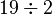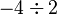# Division by two facts for kids

Kids Encyclopedia Facts

In mathematics, division by two is when a number is divided by two. Some cultures, like the ancient Egyptians, thought this was a different operation than division. Some mathematicians up until the 16th century (1500-1599) thought this too. Today division by two is handled specially in modern computer programming.

Division by two is also called halving, mediation, and dimidiation.

## Binary

In a binary number system, there are only two digits: 0 and 1. All other numbers are represented with those two digits. For example, "one" is 1, "two" is 10, "three" is 11, "four" is 100, and so on. Most of the time people use a number system with ten digits (the numbers 0 through 9.) This is called the decimal number system.

Division by two in binary is very easy. It is done by dropping the last digit on the right of the number. This is called a "bit shift operation." For example, if we performed a bit shift operation on the binary number 100, we'd get 10. Since binary 100 is decimal 4, and binary 10 is decimal 2, this makes sense.

Another example is performing a bit shift operation on 1101. This would leave us with 110, but we dropped a 1 from the end, not a zero. This also makes sense because 1101 in binary is 13 in decimal. If we divide 13 by 2, we get 6 with a remainder of 1 (we have 1 left over.)

### Computers

In computer programming, the symbol `>>` is sometimes used to show a bit shift operation. In Java, we can ask the computer to do the problem$19\div2$ by writing `19 >> 2`. This is the same as writing `19/2`. Both of these versions will give us the answer `9`. There is a problem when trying to do something a problem like$-4\div2$. In Java, if we write `-3/2`, the computer will tell us the answer is `-1`. But if we try to do `-3 >> 2`, the computer will say the answer is `-2`. This will happen every time we try to do a bit shift operation with one negative number. The reason for this is complicated, and has to do with the way negative binary numbers are saved by the computer.